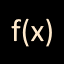#Hef.Math.Interpreter 1.1.1

.NET Framework
`Install-Package Hef.Math.Interpreter -Version 1.1.1`
`dotnet add package Hef.Math.Interpreter --version 1.1.1`
`<PackageReference Include="Hef.Math.Interpreter" Version="1.1.1" />`
For projects that support PackageReference, copy this XML node into the project file to reference the package.
`paket add Hef.Math.Interpreter --version 1.1.1`
`#r "nuget: Hef.Math.Interpreter, 1.1.1"`
#r directive can be used in F# Interactive, C# scripting and .NET Interactive. Copy this into the interactive tool or source code of the script to reference the package.
```// Install Hef.Math.Interpreter as a Cake Addin

// Install Hef.Math.Interpreter as a Cake Tool
#tool nuget:?package=Hef.Math.Interpreter&version=1.1.1```

#### Prerequisites

This software is based on the .Net 2.0 Framework and has no other dependencies.

#### Examples

The interpreter accepts two notation styles. Operations can be written as functions with parenthesis and arguments, or like regular operations with a symbol. There is actually no difference between functions and symbols in the implementation. For instance, the addition can be written `add(1, 2)` or `1 + 2`. Or even `add 1 2` or `+(1, 2)` if you like it.

The complete list of handled operations is availablable at Annex - Handled Operations.

Here is a simple example.

``````Interpreter interpreter = new Interpreter();
double result = interpreter.Calculate("sqrt(4) + 2"); // -> 4
``````

The following example highlights the use of manually registered local and global variables.

``````Interpreter interpreter = new Interpreter();
Interpreter.SetGlobalVar("foo", 1d);
interpreter.SetVar("bar", 2d);
double result = interpreter.Calculate("(\$foo + \$bar) * 2"); // -> 6
``````

The following example highlights the use of `Hef.Math.IInterpreterContext`, that allows the interpreter to access variables provided by other objects.

``````Interpreter interpreter = new Interpreter();
interpreter.SetContext("player", new Player()));
double result = interpreter.Calculate("\$player.level - 1"); // -> 9

class Player : Hef.Math.IInterpreterContext
{
private int level = 10;

public bool TryGetVariable(string name, out double value)
{
value = 0d;

if (name == "level")
{
value = this.level;
return true;
}

return false;
}
}
``````

Each time a formula is calculated, the interpreter has to breaks the formula into nodes and build a tree of operations. This is a time-consumming process.

In order to make it faster, each time a new formula is processed, the intrepreder will keep the generated tree in memory (up to 64). So if the same formula is used again, the tree will be reused, and only the mathematical operations will be recomputed.

If for some reason the cache has to be manually cleared, the `Interpreter` provides a function to do so.

``````Interpreter.ForceClearCache();
``````
Product Versions
.NET Framework net
Compatible target framework(s)

This package has no dependencies.

### NuGet packages

This package is not used by any NuGet packages.

### GitHub repositories

This package is not used by any popular GitHub repositories.